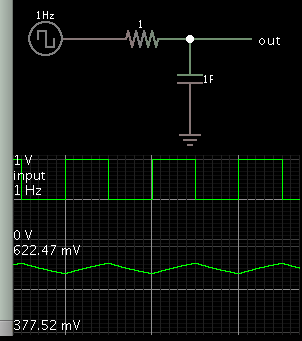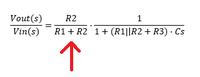# low pass filter analysis

1. ## low pass filter analysis

I have a circuit of low pass filter. the input is 0 to 2.5 Volt. i need the output is 1 volt as DC signal. and I need the values of the R1, R2, R3 and C1. How much should be?

http://obrazki.elektroda.pl/3183955600_1454171405.jpg

M_kuty•

2. ## Re: low pass filter analysis

R3-C1 create a triangle wave riding a DC component. For the RC time constant, it should be much longer than the period of incoming pulses.

R1-R2 form a voltage divider. I think you only need slight voltage reduction, if any. Therefore I think R1 should be small, R2 should be large.

- - - Updated - - -

Think of R1-R2 as a potentiometer. Dial it until you get 1V output.•

3. ## Re: low pass filter analysis

Average value of the input voltage is 0.25*2.5V = 0.625 V, respectively you can't get more than this DC level without an amplifier. 1 V can't be achieved.

To be able to calculate a low pass time constant, it's necessary to specify input frequency and acceptable residual output ripple.•

4. ## Re: low pass filter analysis

if I change the duty cycle to 75%. what will be the average value of the input?
how can the input frequency calculate ?5. ## Re: low pass filter analysisOriginally Posted by FvMAverage value of the input voltage is 0.25*2.5V = 0.625 V, respectively you can't get more than this DC level without an amplifier. 1 V can't be achieved.

To be able to calculate a low pass time constant, it's necessary to specify input frequency and acceptable residual output ripple.
2 questions:

1)DC voltage is average voltage not RMS value? The RMS value of an AC supply is the steady DC which would convert electrical energy into thermal energy at the same rate in a resistance. So if we had at the output a resistance, why use average instead of RMS value? DC = average? RMS acts like beeing DC voltage too...

2)"About the ripple": Is not the output a square wave too? If there is any ripple, give me R and C values for a simple RC low pass filter to simulate it (not because I do not believe you, because I can not find R and C values to see that ripple at the output).

if I change the duty cycle to 75%. what will be the average value of the input?
average input voltage=0.75*2.5 V

how can the input frequency calculate ?•

6. ## Re: low pass filter analysis

The steps are:

at the junction of R1:R2:R3, you see a voltage source with series resistance R1 and parallel resistance R2.

The voltage at this junction will be Vin*R1/(R1+R2). This is input to the R3:C1 low pass filter with time constant R3*C1.

Actually you need to add to R3 the resistance of the source (R1 in series and R2 in parallel).

Your low pass filter output will be always less than 2.5V*0.25 duty cycle.

You choose C1 depending on the desired 3db cut-off point.

- - - Updated - - -Originally Posted by CataM2)"About the ripple": Is not the output a square wave too? If there is any ripple, give me R and C values for a simple RC low pass filter to simulate it (not because I do not believe you, because I can not find R and C values to see that ripple at the output).
In absence of C1, the output will be a square wave with the amplitude and source impedance changed. When you insert C1, it will integrate the voltage and the output will look more like triangular wave (depends on the value of C1 and the input frequency). With very large value of C1 and with a high enough frequency, you will get a stable DC value.

1 members found this post helpful.7. ## Re: low pass filter analysis

I hope this simulation is not cheating (in regard to the exercise questions, that is).

It answers several questions in this thread. It demonstrates behavior of RC time constant=1, with a frequency of 1 Hz.

1 Hz square wave
1 F
1 ohmBy some mathematical quirk, the capacitor points out the definition of the RC time constant. It reaches 63% of applied voltage after one time constant.

1 members found this post helpful.8. ## Re: low pass filter analysis

The full transfer function of the filter showed by the OP in post #1 is this one:Regarding the term pointed with the arrow, to make it value "1" so then acts like the simple RC low pass filter, it is needed high R2 and low R1 which then changes the time constant etc..

My question is: Is not better to use the simple RC low pass filter rather than the other one?--[[ ]]--